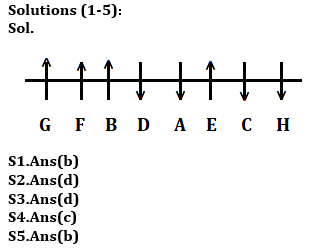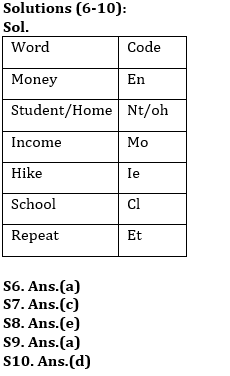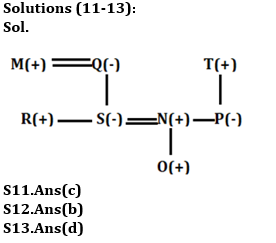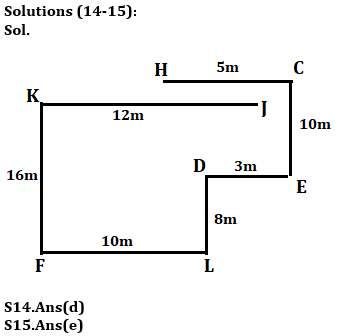Latest Banking jobs   »   Reasoning Ability Quiz For ESIC- UDC,...

# Reasoning Ability Quiz For ESIC- UDC, Steno, MTS Prelims 2022- 17th January

Directions (1-5): Study the information carefully and answer the questions given below.
Eight-person A, B, C, D, E, F, G and H are sitting in a straight line. Some persons are facing south and some persons are facing north.
D sits second to the left of E, who sits second to the right of H. D sits third to the right of G, who sits at one of the extreme ends. G faces north direction. B sits immediate right of F. E is not immediate neighbor of G. B sits fourth to the right of C. Immediate neighbor of D faces opposite directions. F sits second to the right of D, who sits immediate right of B. Persons sit at the extreme ends faces opposite direction to each other.

Q1. How many persons are facing south?
(a) One
(b) Four
(c) More than Four
(d) Three
(e) None

Q2. Who among the following person sit immediate right of G?
(a) B
(b) D
(c) A
(d) F
(e) None of these

Q3. What is the position of H with respect to B?
(a) Second to the right
(b) Fifth to the left
(c) Fourth to the right
(d) Fifth to the right
(e) Fourth to the left

Q4. Four of the following five are alike in certain way based from a group, find the one that does not belong to that group?
(a) B
(b) F
(c) H
(d) G
(e) E

Q5. How many persons sit between E and G?
(a) One
(b) Four
(c) None
(d) Three
(e) Two

Directions (6-10): Study the following information carefully and answer the questions given below:
In a certain code language:
‘Student Home Money’ is written as ‘nt oh en’,
‘Money Income hike’ is written as ‘en mo ie’,
‘Home School Student’ is written as ‘nt oh cl’
‘Repeat Income Money’ is written as ‘mo en et’.

Q6. What is the code for ‘Income Repeat’ in the given code language?
(a) mo et
(b) et oh
(c) nt et
(d) mo nt
(e) None of these

Q7. What is the code for ‘Hike’ in the given code language?
(a) nt
(b) oh
(c) ie
(d) cl
(e) None of these

Q8. What is the code for ‘Student’ in the given code language?
(a) nt
(b) cl
(c) oh
(d) et
(e) Can’t be determined

Q9. What is the code for ‘money school’ in the given code language?
(a) en cl
(b) et nt
(c) nt oh
(d) ie et
(e) None of these

Q10. What may be the code for ‘Home Science’?
(a) oh en
(b) ie nt
(c) er cl
(d) nt er
(e) None of these

Directions (11-13): Study the following information carefully and answer the questions given below:
There are eight members i.e. M, N, O, P, Q, R, S and T who belong to a family having three generation.
N and P are siblings. P is the only daughter of T and she is unmarried. O is the grandson of Q. T is the father-in-law of S. R is the son of M, who is husband of Q. R is the brother of S.

Q11. How is N related to O?
(a) Brother
(b) Son
(c) Father
(d) Mother
(e) None of these

Q12. Who among the following is the maternal grandfather of O?
(a) T
(b) M
(c) Q
(d) R
(e) Can’t be determined

Q13. How S related to T?
(a) Son
(b) Daughter
(c) Son-in-law
(d) Daughter-in-law
(e) None of these

Directions (14-15): Study the given information and answer the following question.
Point J is 12m east of point K. Point H is 5m west of point C. Point L is 10m east of Point F. Point F is 16m south of point K. Point D is 8m north of point L. Point E is 3m east of point D. Point C is 10m north of point E.

Q14. What is the shortest distance between H and D?
(a) 10m
(b) 11m
(c) 9m
(d) √104 m
(e) None of these

Q15. Point L is in which direction with respect to C?
(a) South
(b) North-East
(c) East
(d) North-West
(e) South-West

Solutions#### Congratulations!Union Budget 2023-24: Free PDF